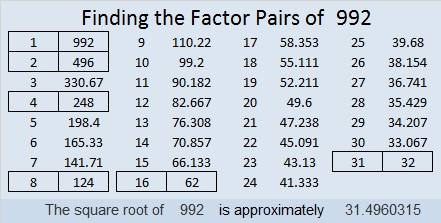# 992 Christmas Factor Tree

Artificial Christmas trees have to be assembled. Sometimes the assembly is easy, and sometimes it is frustrating.

This Christmas tree puzzle can be solved using LOGIC and an ordinary multiplication table, but there’s a good chance it will frustrate you. Go ahead and try to solve it!Print the puzzles or type the solution in this excel file: 10-factors-986-992

The number 992 also can make a nice looking, well-balanced factor tree:992 is the product of two consecutive numbers: 31 × 32 = 992.
Because of that fact, 992 is the sum of the first 31 EVEN numbers:
2 + 4 + 6 + 8 + 10 + . . . + 54 + 56 + 58 + 60 + 62 = 992

992 is palindrome 212 in BASE 22 because 2(22²) + 1(22) + 2(1) = 922. That was a lot of 2’s and 1’s in that fun fact!

• 992 is a composite number.
• Prime factorization: 992 = 2 × 2 × 2 × 2 × 2 × 31, which can be written 992 = 2⁵ × 31
• The exponents in the prime factorization are 5 and 1. Adding one to each and multiplying we get (5 + 1)(1 + 1) = 6 × 2 = 12. Therefore 992 has exactly 12 factors.
• Factors of 992: 1, 2, 4, 8, 16, 31, 32, 62, 124, 248, 496, 992
• Factor pairs: 992 = 1 × 992, 2 × 496, 4 × 248, 8 × 124, 16 × 62, or 31 × 32
• Taking the factor pair with the largest square number factor, we get √992 = (√16)(√62) = 4√62 ≈ 31.49603